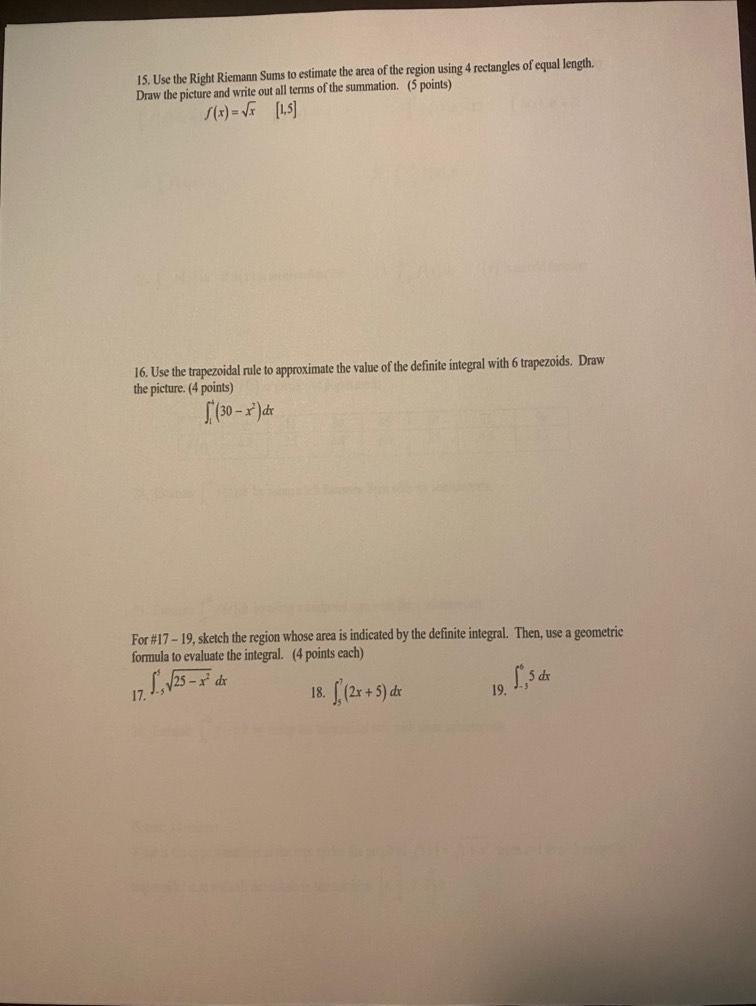Question:

# 15. Use the Right Riemann Sums to estimate the area of the region using 4 rectangles of equal length. Draw the picture and write15. Use the Right Riemann Sums to estimate the area of the region using 4 rectangles of equal length. Draw the picture and write out all terms of the summation. (5 points) S(x) = V [1,5] 16. Use the trapezoidal rule to approximate the value of the definite integral with 6 trapezoids. Draw the picture. (4 points) [(30 - r ) de For#17 - 19, sketch the region whose area is indicated by the definite integral. Then, use a geometric formula to evaluate the integral. (4 points each) L, /25 - x de 18. ((2x + 5) de 19. 1,5 17